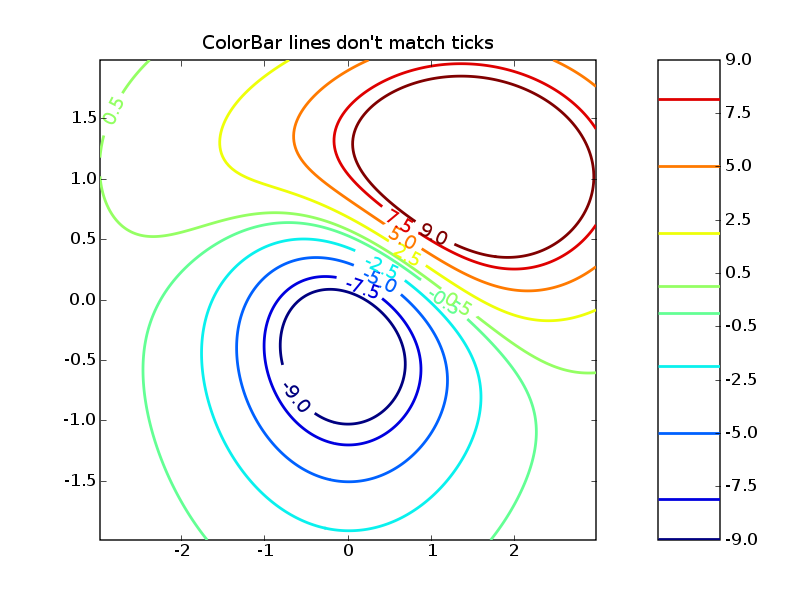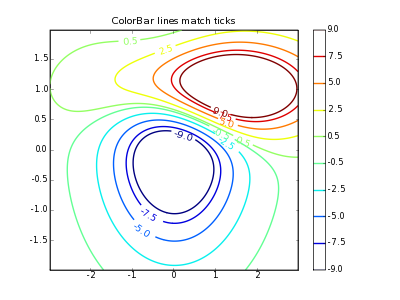# Trouble with colorbar cspacing='linear'

Hello,

I am trying to construct a contour plot where the contours are
separated by a constant amount but I would like a color bar (or
something) to show what the values of the different levels are. I will
have too many contours for clabels to be effective.

When I use colorbar with unequally spaced contours and use
cspacing=‘linear’ the ticks are spaced nicely but the colors are not.

The following code exemplifies the problem:

``````*#!/usr/bin/python*
from pylab import *
*#Compute Data*
delta = 0.025
x = arange(-3.0, 3.0, delta)
y = arange(-2.0, 2.0, delta)
X, Y = meshgrid(x, y)
Z1 = bivariate_normal(X, Y, 1.0, 1.0, 0.0, 0.0)
Z2 = bivariate_normal(X, Y, 1.5, 0.5, 1, 1)
*# difference of Gaussians*
Z = 100.0 * (Z2 - Z1)
*#Draw figure*
figure()
levels=[-9,-7.5,-5,-2.5,-0.5,0.5,2.5,5,7.5,9]
CS = contour(             Z, levels,
origin='lower'             ,
linewidths=2             ,
extent=(-3,3,-2,2))
clabel(       CS,
inline=1       ,
fmt='%1.1f'       ,
fontsize=14)
colorbar(CS,cspacing='linear')  *# make a colorbar for the contour lines*
title("ColorBar lines don't match ticks")
show()
``````

I have attached the file ‘BadColorBar.png’

Any help would be appreciated,

Karl EdlerKarl Edler wrote:

Hello,

I am trying to construct a contour plot where the contours are separated by a constant amount but I would like a color bar (or something) to show what the values of the different levels are. I will have too many contours for clabels to be effective.

When I use colorbar with unequally spaced contours and use cspacing='linear' the ticks are spaced nicely but the colors are not.

Karl,

This was fixed some time ago with completely new colorbar code, including a change in the API. Attached are a modification of your test program to suit the API (and make a smaller png file for this email), and the result.

Ericlinecbar.py (690 Bytes)